# Creating Custom Estimators

This document introduces custom Estimators. In particular, this document demonstrates how to create a custom `tf.estimator.Estimator` that mimics the behavior of the pre-made Estimator `tf.estimator.DNNClassifier` in solving the Iris problem. See the Pre-Made Estimators chapter for details on the Iris problem.

To download and access the example code invoke the following two commands:

``````git clone https://github.com/tensorflow/models/
cd models/samples/core/get_started
``````

In this document we will be looking at `custom_estimator.py`. You can run it with the following command:

``````python custom_estimator.py
``````

If you are feeling impatient, feel free to compare and contrast `custom_estimator.py` with `premade_estimator.py`. (which is in the same directory).

## Pre-made vs. custom

As the following figure shows, pre-made Estimators are subclasses of the `tf.estimator.Estimator` base class, while custom Estimators are an instance of tf.estimator.Estimator: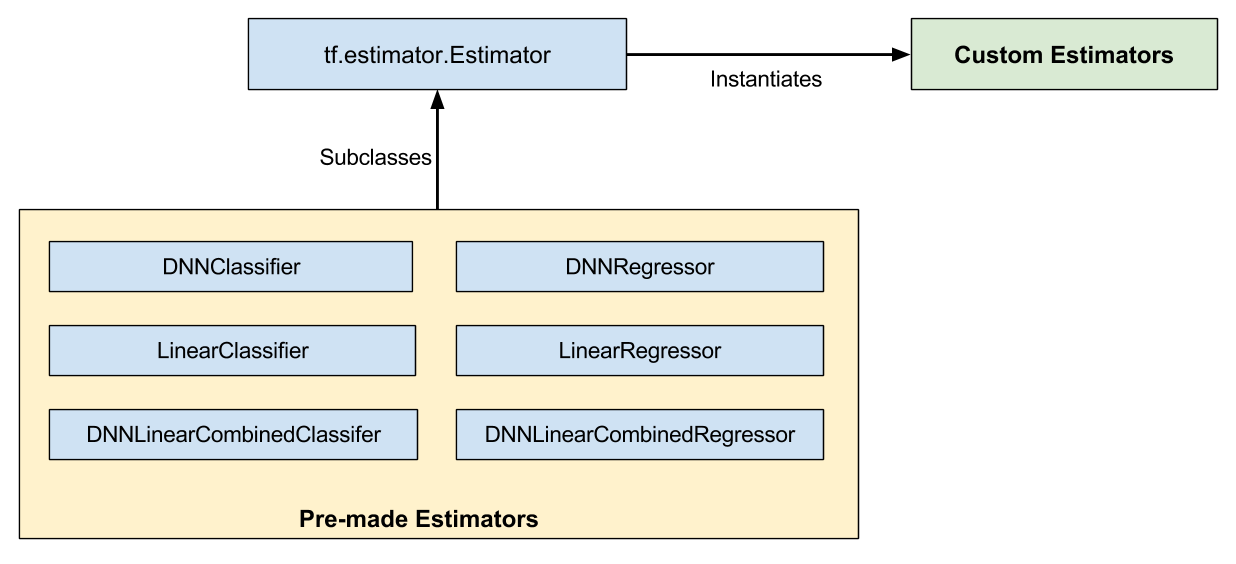Pre-made and custom Estimators are all Estimators.

Pre-made Estimators are fully baked. Sometimes though, you need more control over an Estimator's behavior. That's where custom Estimators come in. You can create a custom Estimator to do just about anything. If you want hidden layers connected in some unusual fashion, write a custom Estimator. If you want to calculate a unique metric for your model, write a custom Estimator. Basically, if you want an Estimator optimized for your specific problem, write a custom Estimator.

A model function (or `model_fn`) implements the ML algorithm. The only difference between working with pre-made Estimators and custom Estimators is:

• With pre-made Estimators, someone already wrote the model function for you.
• With custom Estimators, you must write the model function.

Your model function could implement a wide range of algorithms, defining all sorts of hidden layers and metrics. Like input functions, all model functions must accept a standard group of input parameters and return a standard group of output values. Just as input functions can leverage the Dataset API, model functions can leverage the Layers API and the Metrics API.

Let's see how to solve the Iris problem with a custom Estimator. A quick reminder--here's the organization of the Iris model that we're trying to mimic: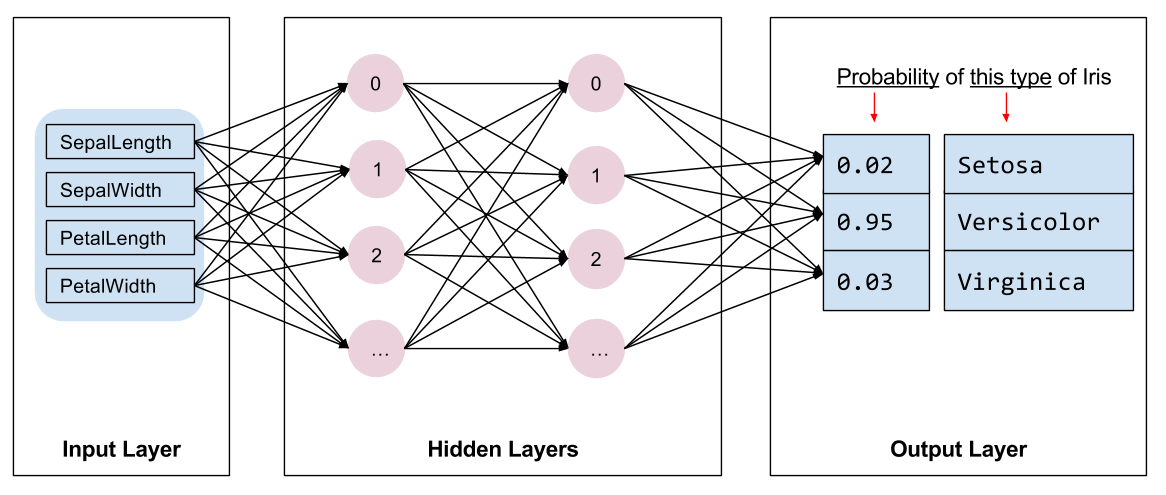Our implementation of Iris contains four features, two hidden layers, and a logits output layer.

## Write an Input function

Our custom Estimator implementation uses the same input function as our pre-made Estimator implementation, from `iris_data.py`. Namely:

``````def train_input_fn(features, labels, batch_size):
"""An input function for training"""
# Convert the inputs to a Dataset.
dataset = tf.data.Dataset.from_tensor_slices((dict(features), labels))

# Shuffle, repeat, and batch the examples.
dataset = dataset.shuffle(1000).repeat().batch(batch_size)

# Return the dataset.
return dataset
``````

This input function builds an input pipeline that yields batches of `(features, labels)` pairs, where `features` is a dictionary features.

## Create feature columns

As detailed in the Premade Estimators and Feature Columns chapters, you must define your model's feature columns to specify how the model should use each feature. Whether working with pre-made Estimators or custom Estimators, you define feature columns in the same fashion.

The following code creates a simple `numeric_column` for each input feature, indicating that the value of the input feature should be used directly as an input to the model:

``````# Feature columns describe how to use the input.
my_feature_columns = []
for key in train_x.keys():
my_feature_columns.append(tf.feature_column.numeric_column(key=key))
``````

## Write a model function

The model function we'll use has the following call signature:

``````def my_model_fn(
features, # This is batch_features from input_fn
labels,   # This is batch_labels from input_fn
mode,     # An instance of tf.estimator.ModeKeys
params):  # Additional configuration
``````

The first two arguments are the batches of features and labels returned from the input function; that is, `features` and `labels` are the handles to the data your model will use. The `mode` argument indicates whether the caller is requesting training, predicting, or evaluation.

The caller may pass `params` to an Estimator's constructor. Any `params` passed to the constructor are in turn passed on to the `model_fn`. In `custom_estimator.py` the following lines create the estimator and set the params to configure the model. This configuration step is similar to how we configured the `tf.estimator.DNNClassifier` in Premade Estimators.

``````classifier = tf.estimator.Estimator(
model_fn=my_model_fn,
params={
'feature_columns': my_feature_columns,
# Two hidden layers of 10 nodes each.
'hidden_units': [10, 10],
# The model must choose between 3 classes.
'n_classes': 3,
})
``````

To implement a typical model function, you must do the following:

## Define the model

The basic deep neural network model must define the following three sections:

### Define the input layer

The first line of the `model_fn` calls `tf.feature_column.input_layer` to convert the feature dictionary and `feature_columns` into input for your model, as follows:

``````    # Use `input_layer` to apply the feature columns.
net = tf.feature_column.input_layer(features, params['feature_columns'])
``````

The preceding line applies the transformations defined by your feature columns, creating the model's input layer.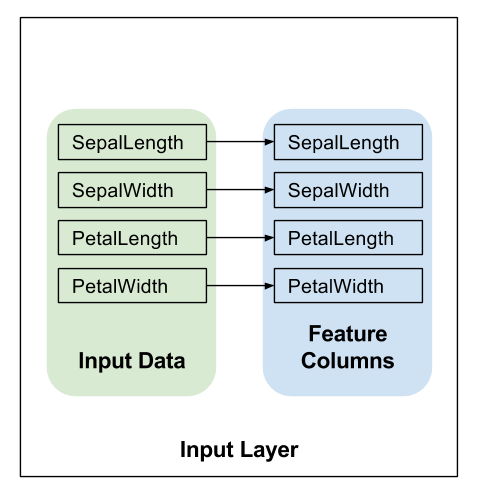### Hidden Layers

If you are creating a deep neural network, you must define one or more hidden layers. The Layers API provides a rich set of functions to define all types of hidden layers, including convolutional, pooling, and dropout layers. For Iris, we're simply going to call `tf.layers.dense` to create hidden layers, with dimensions defined by `params['hidden_layers']`. In a `dense` layer each node is connected to every node in the preceding layer. Here's the relevant code:

``````    # Build the hidden layers, sized according to the 'hidden_units' param.
for units in params['hidden_units']:
net = tf.layers.dense(net, units=units, activation=tf.nn.relu)
``````
• The `units` parameter defines the number of output neurons in a given layer.
• The `activation` parameter defines the activation functionRelu in this case.

The variable `net` here signifies the current top layer of the network. During the first iteration, `net` signifies the input layer. On each loop iteration `tf.layers.dense` creates a new layer, which takes the previous layer's output as its input, using the variable `net`.

After creating two hidden layers, our network looks as follows. For simplicity, the figure does not show all the units in each layer.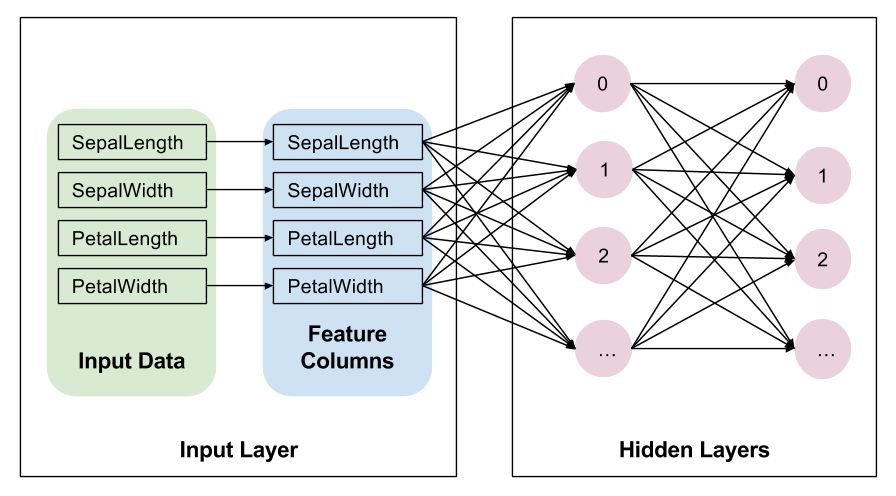Note that `tf.layers.dense` provides many additional capabilities, including the ability to set a multitude of regularization parameters. For the sake of simplicity, though, we're going to simply accept the default values of the other parameters.

### Output Layer

We'll define the output layer by calling `tf.layers.dense` yet again, this time without an activation function:

``````    # Compute logits (1 per class).
logits = tf.layers.dense(net, params['n_classes'], activation=None)
``````

Here, `net` signifies the final hidden layer. Therefore, the full set of layers is now connected as follows: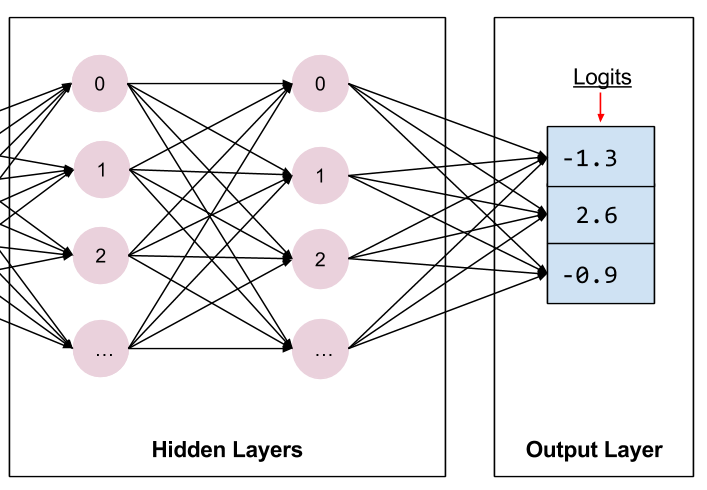The final hidden layer feeds into the output layer.

When defining an output layer, the `units` parameter specifies the number of outputs. So, by setting `units` to `params['n_classes']`, the model produces one output value per class. Each element of the output vector will contain the score, or "logit", calculated for the associated class of Iris: Setosa, Versicolor, or Virginica, respectively.

Later on, these logits will be transformed into probabilities by the `tf.nn.softmax` function.

## Implement training, evaluation, and prediction

The final step in creating a model function is to write branching code that implements prediction, evaluation, and training.

The model function gets invoked whenever someone calls the Estimator's `train`, `evaluate`, or `predict` methods. Recall that the signature for the model function looks like this:

``````def my_model_fn(
features, # This is batch_features from input_fn
labels,   # This is batch_labels from input_fn
mode,     # An instance of tf.estimator.ModeKeys, see below
params):  # Additional configuration
``````

Focus on that third argument, mode. As the following table shows, when someone calls `train`, `evaluate`, or `predict`, the Estimator framework invokes your model function with the mode parameter set as follows:

For example, suppose you instantiate a custom Estimator to generate an object named `classifier`. Then, you make the following call:

``````classifier = tf.estimator.Estimator(...)
classifier.train(input_fn=lambda: my_input_fn(FILE_TRAIN, True, 500))
``````

The Estimator framework then calls your model function with mode set to `ModeKeys.TRAIN`.

Your model function must provide code to handle all three of the mode values. For each mode value, your code must return an instance of `tf.estimator.EstimatorSpec`, which contains the information the caller requires. Let's examine each mode.

### Predict

When the Estimator's `predict` method is called, the `model_fn` receives `mode = ModeKeys.PREDICT`. In this case, the model function must return a `tf.estimator.EstimatorSpec` containing the prediction.

The model must have been trained prior to making a prediction. The trained model is stored on disk in the `model_dir` directory established when you instantiated the Estimator.

The code to generate the prediction for this model looks as follows:

``````# Compute predictions.
predicted_classes = tf.argmax(logits, 1)
if mode == tf.estimator.ModeKeys.PREDICT:
predictions = {
'class_ids': predicted_classes[:, tf.newaxis],
'probabilities': tf.nn.softmax(logits),
'logits': logits,
}
return tf.estimator.EstimatorSpec(mode, predictions=predictions)
``````

The prediction dictionary contains everything that your model returns when run in prediction mode.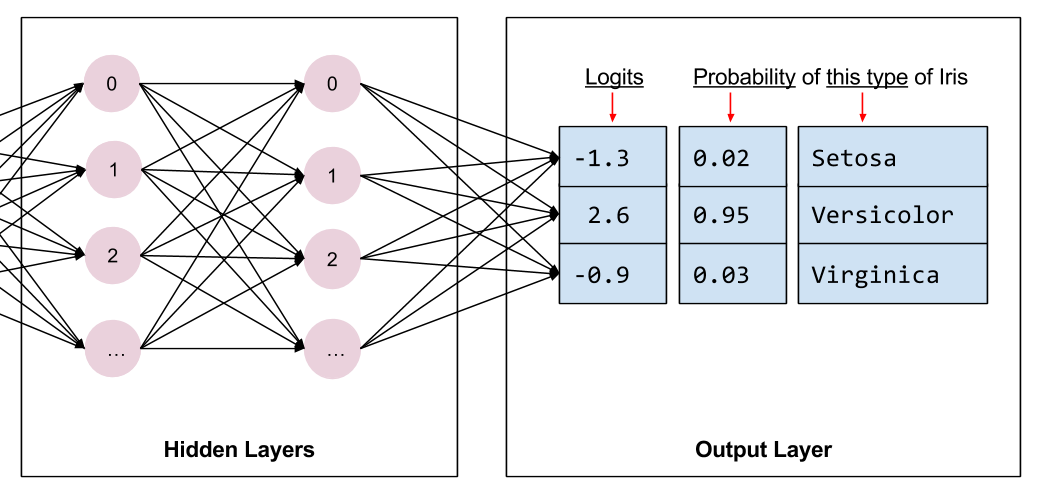The `predictions` holds the following three key/value pairs:

• `class_ids` holds the class id (0, 1, or 2) representing the model's prediction of the most likely species for this example.
• `probabilities` holds the three probabilities (in this example, 0.02, 0.95, and 0.03)
• `logit` holds the raw logit values (in this example, -1.3, 2.6, and -0.9)

We return that dictionary to the caller via the `predictions` parameter of the `tf.estimator.EstimatorSpec`. The Estimator's `tf.estimator.Estimator.predict` method will yield these dictionaries.

### Calculate the loss

For both training and evaluation we need to calculate the model's loss. This is the objective that will be optimized.

We can calculate the loss by calling `tf.losses.sparse_softmax_cross_entropy`. The value returned by this function will be approximately 0 at lowest, when the probability of the correct class (at index `label`) is near 1.0. The loss value returned is progressively larger as the probability of the correct class decreases.

This function returns the average over the whole batch.

``````# Compute loss.
loss = tf.losses.sparse_softmax_cross_entropy(labels=labels, logits=logits)
``````

### Evaluate

When the Estimator's `evaluate` method is called, the `model_fn` receives `mode = ModeKeys.EVAL`. In this case, the model function must return a `tf.estimator.EstimatorSpec` containing the model's loss and optionally one or more metrics.

Although returning metrics is optional, most custom Estimators do return at least one metric. TensorFlow provides a Metrics module `tf.metrics` to calculate common metrics. For brevity's sake, we'll only return accuracy. The `tf.metrics.accuracy` function compares our predictions against the true values, that is, against the labels provided by the input function. The `tf.metrics.accuracy` function requires the labels and predictions to have the same shape. Here's the call to `tf.metrics.accuracy`:

``````# Compute evaluation metrics.
accuracy = tf.metrics.accuracy(labels=labels,
predictions=predicted_classes,
name='acc_op')
``````

The `tf.estimator.EstimatorSpec` returned for evaluation typically contains the following information:

• `loss`, which is the model's loss
• `eval_metric_ops`, which is an optional dictionary of metrics.

So, we'll create a dictionary containing our sole metric. If we had calculated other metrics, we would have added them as additional key/value pairs to that same dictionary. Then, we'll pass that dictionary in the `eval_metric_ops` argument of `tf.estimator.EstimatorSpec`. Here's the code:

``````metrics = {'accuracy': accuracy}
tf.summary.scalar('accuracy', accuracy)

if mode == tf.estimator.ModeKeys.EVAL:
return tf.estimator.EstimatorSpec(
mode, loss=loss, eval_metric_ops=metrics)
``````

The `tf.summary.scalar` will make accuracy available to TensorBoard in both `TRAIN` and `EVAL` modes. (More on this later).

### Train

When the Estimator's `train` method is called, the `model_fn` is called with `mode = ModeKeys.TRAIN`. In this case, the model function must return an `EstimatorSpec` that contains the loss and a training operation.

Building the training operation will require an optimizer. We will use `tf.train.AdagradOptimizer` because we're mimicking the `DNNClassifier`, which also uses `Adagrad` by default. The `tf.train` package provides many other optimizers—feel free to experiment with them.

Here is the code that builds the optimizer:

``````optimizer = tf.train.AdagradOptimizer(learning_rate=0.1)
``````

Next, we build the training operation using the optimizer's `tf.train.Optimizer.minimize` method on the loss we calculated earlier.

The `minimize` method also takes a `global_step` parameter. TensorFlow uses this parameter to count the number of training steps that have been processed (to know when to end a training run). Furthermore, the `global_step` is essential for TensorBoard graphs to work correctly. Simply call `tf.train.get_global_step` and pass the result to the `global_step` argument of `minimize`.

Here's the code to train the model:

``````train_op = optimizer.minimize(loss, global_step=tf.train.get_global_step())
``````

The `tf.estimator.EstimatorSpec` returned for training must have the following fields set:

• `loss`, which contains the value of the loss function.
• `train_op`, which executes a training step.

Here's our code to call `EstimatorSpec`:

``````return tf.estimator.EstimatorSpec(mode, loss=loss, train_op=train_op)
``````

The model function is now complete.

## The custom Estimator

Instantiate the custom Estimator through the Estimator base class as follows:

``````    # Build 2 hidden layer DNN with 10, 10 units respectively.
classifier = tf.estimator.Estimator(
model_fn=my_model_fn,
params={
'feature_columns': my_feature_columns,
# Two hidden layers of 10 nodes each.
'hidden_units': [10, 10],
# The model must choose between 3 classes.
'n_classes': 3,
})
``````

Here the `params` dictionary serves the same purpose as the key-word arguments of `DNNClassifier`; that is, the `params` dictionary lets you configure your Estimator without modifying the code in the `model_fn`.

The rest of the code to train, evaluate, and generate predictions using our Estimator is the same as in the Premade Estimators chapter. For example, the following line will train the model:

``````# Train the Model.
classifier.train(
input_fn=lambda:iris_data.train_input_fn(train_x, train_y, args.batch_size),
steps=args.train_steps)
``````

## TensorBoard

You can view training results for your custom Estimator in TensorBoard. To see this reporting, start TensorBoard from your command line as follows:

``````# Replace PATH with the actual path passed as model_dir
tensorboard --logdir=PATH
``````

Then, open TensorBoard by browsing to: http://localhost:6006

All the pre-made Estimators automatically log a lot of information to TensorBoard. With custom Estimators, however, TensorBoard only provides one default log (a graph of the loss) plus the information you explicitly tell TensorBoard to log. For the custom Estimator you just created, TensorBoard generates the following: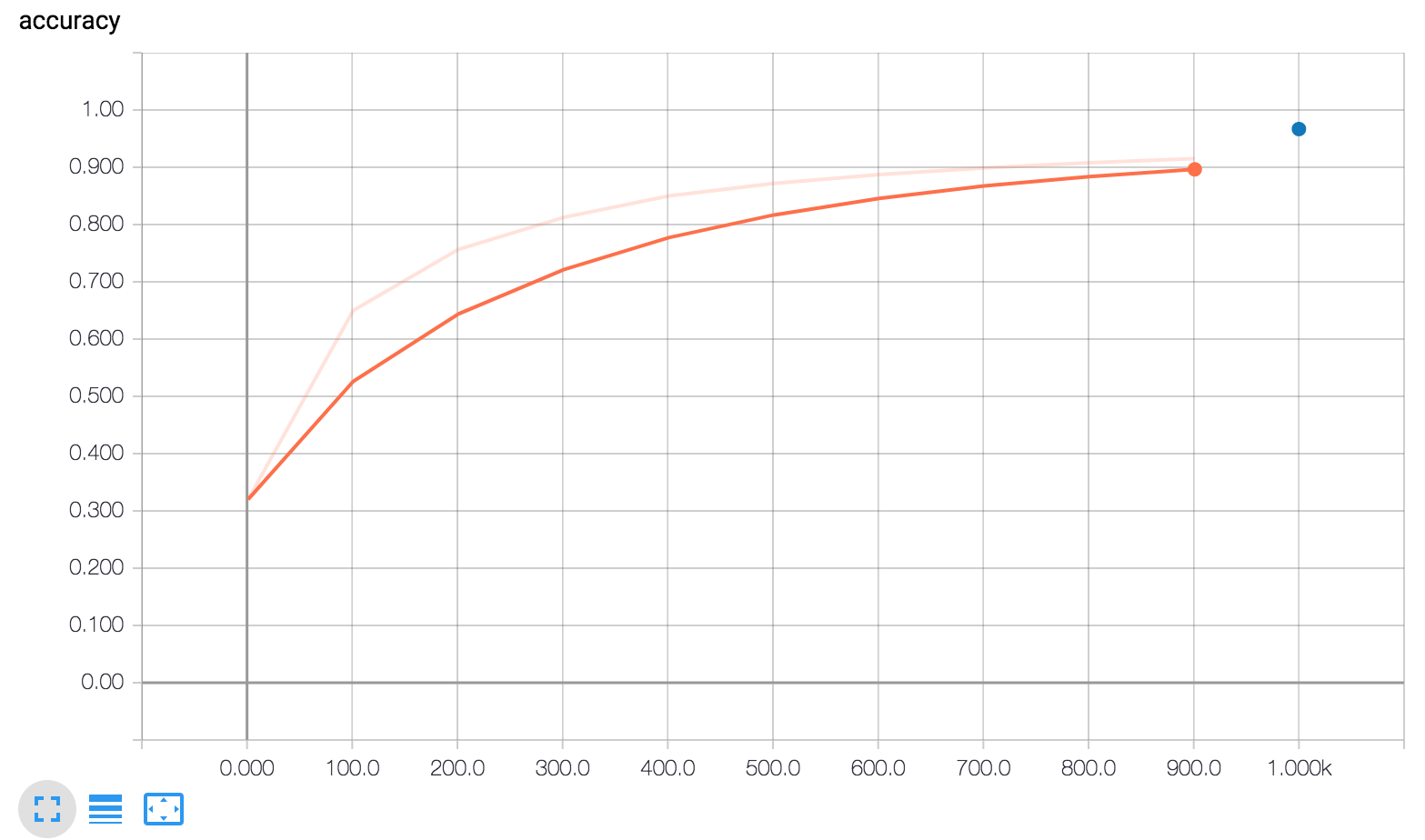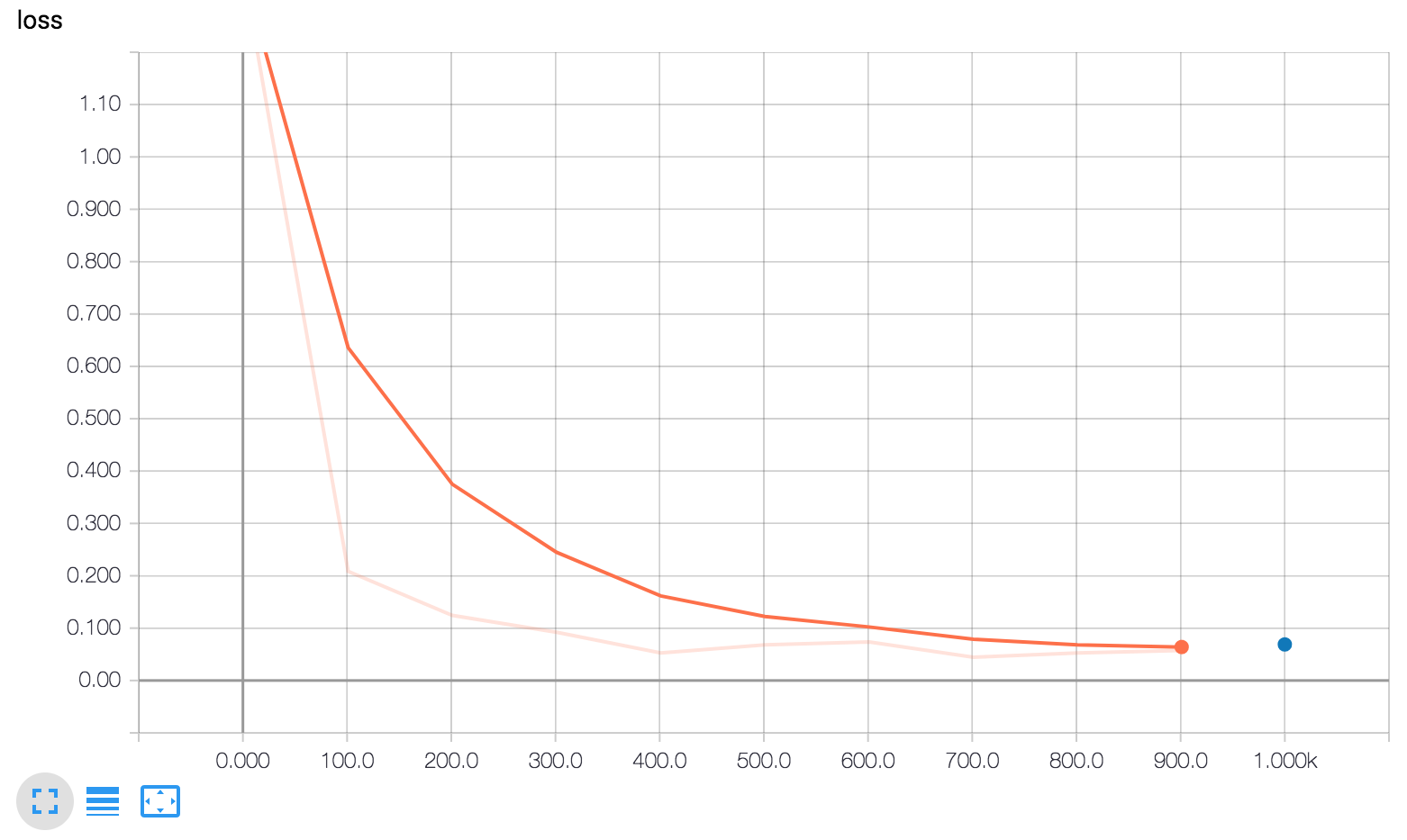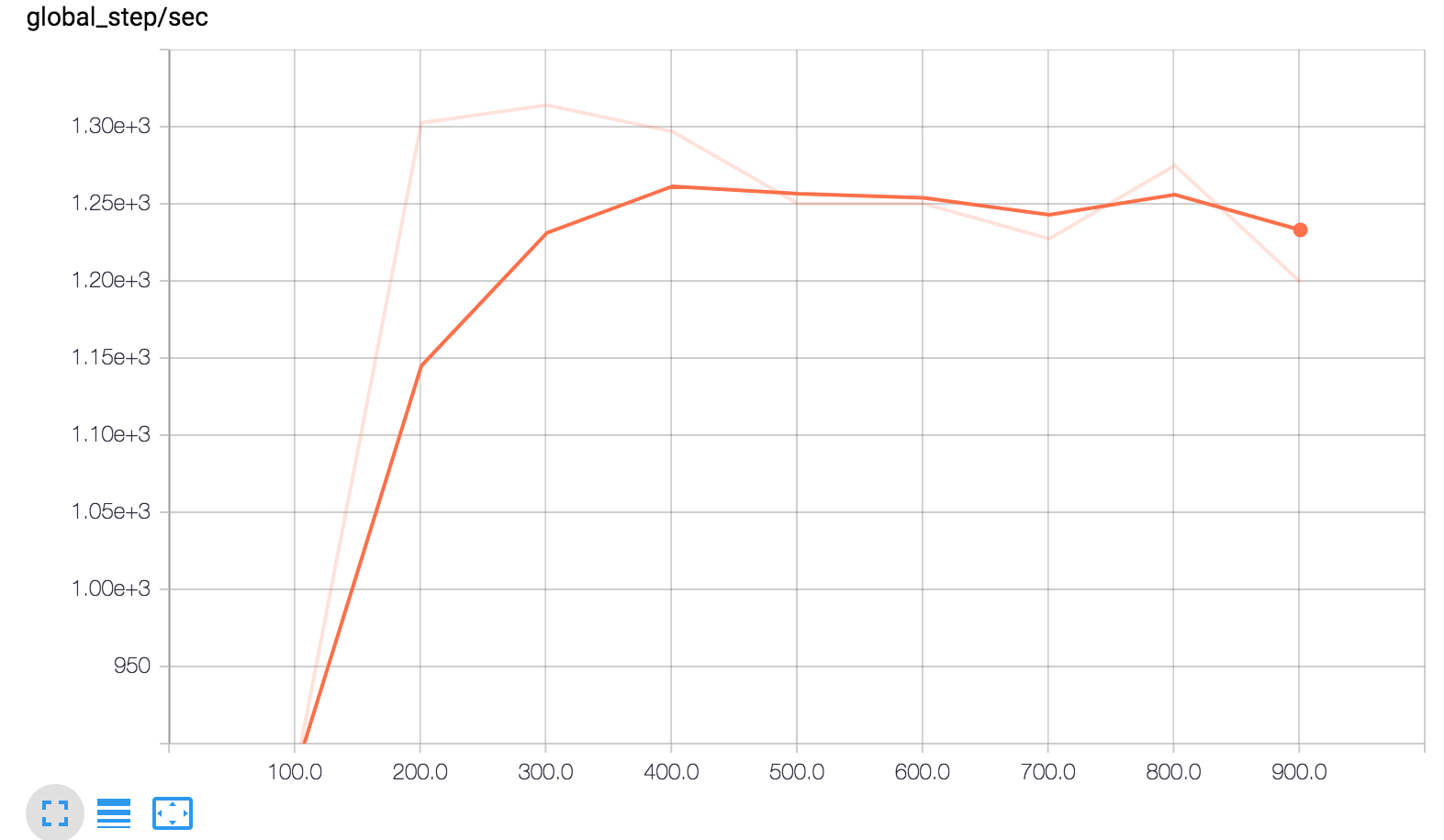TensorBoard displays three graphs.

In brief, here's what the three graphs tell you:

• global_step/sec: A performance indicator showing how many batches (gradient updates) we processed per second as the model trains.

• loss: The loss reported.

• accuracy: The accuracy is recorded by the following two lines:

• `eval_metric_ops={'my_accuracy': accuracy}`, during evaluation.
• `tf.summary.scalar('accuracy', accuracy)`, during training.

These tensorboard graphs are one of the main reasons it's important to pass a `global_step` to your optimizer's `minimize` method. The model can't record the x-coordinate for these graphs without it.

Note the following in the `my_accuracy` and `loss` graphs:

• The orange line represents training.
• The blue dot represents evaluation.

During training, summaries (the orange line) are recorded periodically as batches are processed, which is why it becomes a graph spanning x-axis range.

By contrast, evaluation produces only a single point on the graph for each call to `evaluate`. This point contains the average over the entire evaluation call. This has no width on the graph as it is evaluated entirely from the model state at a particular training step (from a single checkpoint).

As suggested in the following figure, you may see and also selectively disable/enable the reporting using the controls on the left side.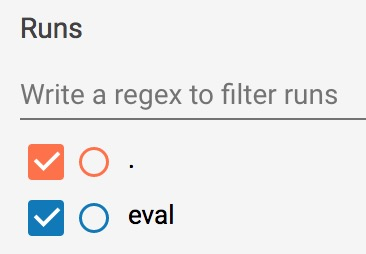Enable or disable reporting.

## Summary

Although pre-made Estimators can be an effective way to quickly create new models, you will often need the additional flexibility that custom Estimators provide. Fortunately, pre-made and custom Estimators follow the same programming model. The only practical difference is that you must write a model function for custom Estimators; everything else is the same.

For more details, be sure to check out: